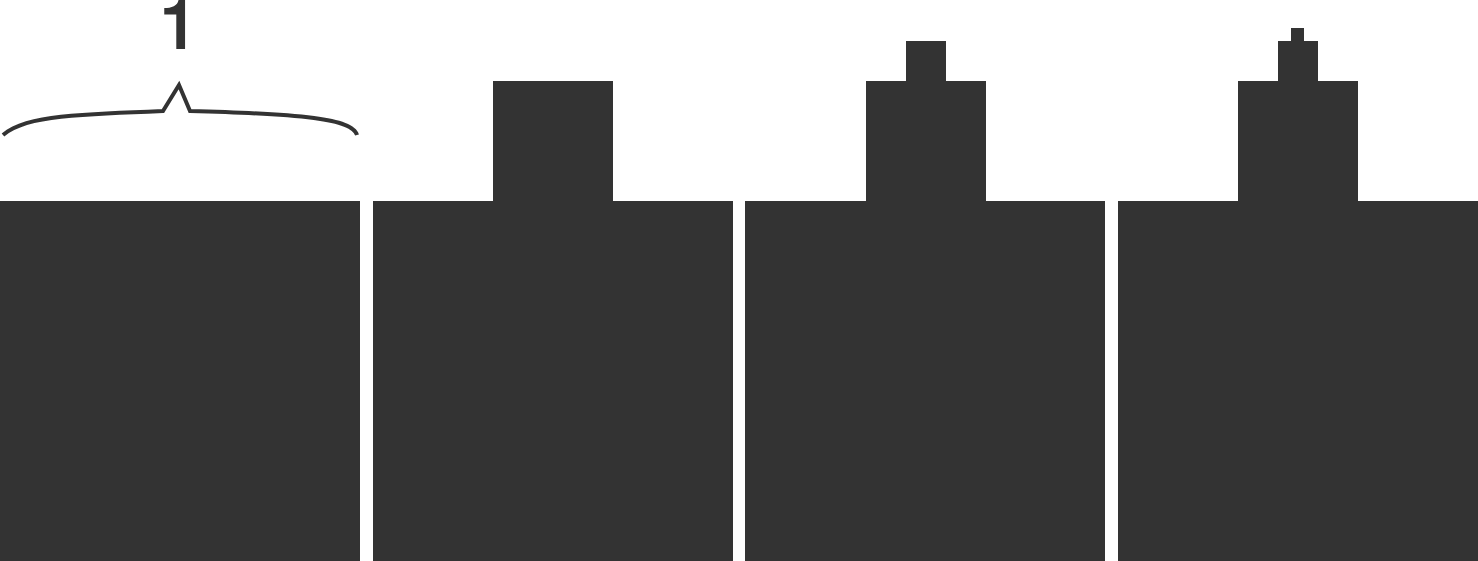# Fractal Fraction

Geometry Level 3

Jim has a unit square.

• On top of this unit square, he places a square with side length equal to $\frac13$ the side length of the square below.
• On top of that square, he places another square which again has side length equal to $\frac13$ the side length of the square below, and so on.
• He repeats this process until he has created an infinite stack of diminishing squares--a fractal.

What is the ratio of the perimeter of the fractal to the area of the fractal?×